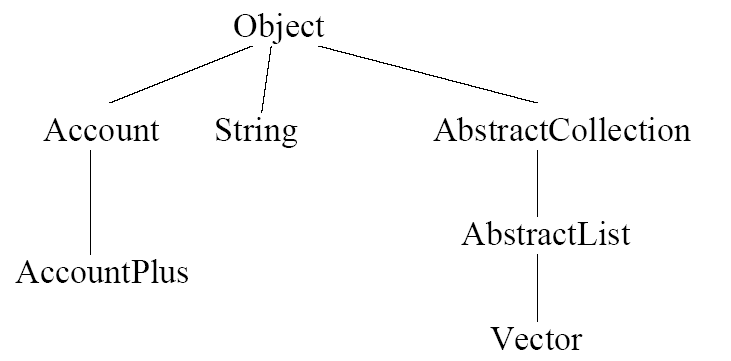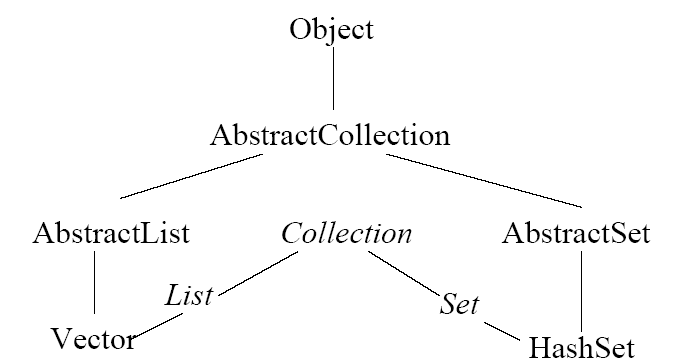Java Notes‎ > ‎

Description

Inheritance allows for efficient reuse of existing programming structures and procedures.

Animals Inheritance Example

1. version 1 (handout (doc)): Basic inheritance using extends, no-args constructor in superclass needed, overloaded constructors. Using classes Animal.java, Dog.javaDriver.java
2. version 2 (handout (doc)): Chaining constructors using this, invoking superclass constructor using super, for Animal2.javaDog2.javaDriver2.java
• Overriding superclass toString method: access specifier (i.e. public) for the overriding class may not be more restrictive than that of the superclass.
• Cannot string more than one super together. (i.e. super.super.toString() is invalid.)
3. version 3 ( handout (doc)): Polymorphism, where the type of an object is determined at runtime and changes the behavior of the program. 3 Different animals all have the sound method implemented.
• For polymorphism to work (e.g. objects of type Animal all call the correct class corresponding to the actual type of each one) the following must be true:
• The method call (e.g. sound() ) must be through an object of the base class (e.g. Animal).
• The method in the derived class must have the same argument signature and return type as that in the base class.
• The method in the derived class must have an access specifier no more restrictive than that of the base class
4. version #4: abstract classes.
• A class with one or more abstract methods is itself an abstract class, which cannot be instantiated. It is useful for organizing subclasses.
• An abstract class method declaration ends with a semi-colon, e.g. method sound() in class Animal4.

All user-defined classes themselves are actually subclasses of super-class Object. Class Object has some public methods which we commonly override:
• toString() - This gives us a representation of an object as a String
• equals() - This compares the calling object with the object sent as a parameter, returning true if they are actually the same object, not just containing the same values.
• getClass() - This returns an object of type Class that identifies the class of the current object.
• We could use this, for instance, if we want to print only elements of class Dog.
• This also illustrates using qualified names to access methods outside the current package.
• hashCode() - This calculates an integer hash code value for an object and is used for storing objects in hash tables.

Bank Account Inheritance Example

See the corresponding class handout (doc)for the Account class.

Consider a Bank Account class and a class representing a transaction:

` class Account  {`

`        String name;`

`        Vector transv;`

`        int balance;`

`        Account(String n) {`

`            transv = new Vector();`

`            balance = 0;`

`            name = n;`

`        }`

`        boolean checkTrans(Trans t) {`

`            return (balance + t.amount >= 0);`

`        }`

`        void post(Trans t) {`

`            transv.add(t);`

`            balance += t.amount;`

`        }`

` }`

`class Trans {`

`        int amount;`

`        Date date;`

`        //...`

`}`

Note that transactions can be checked to ensure balance > 0.  Class Account can be extended by inheritance using the extends keyword:

`class AccountPlus extends Account {`

`        int creditLimit;`

`        AccountPlus(String n, int c) {`

`            super(n);`

`            creditLimit = c;`

`        }`

`        boolean checkTrans(Trans t) {`

`            return (balance + creditLimit + t.amount >= 0);`

`        }`

`    }`

Note that there is a new field creditLimit, and method checkTrans( Trans t) overrides the method of the same name in the super class.  .  At runtime the system will decide which version of these two methods to call.  Account is the superclass of AccountPlus.  A variable at runtime can refer to an object not of its type as long as it is a subclass.

Sometimes the code clearly indicates the type of a variable:

`AccountPlus acc = new AccountPlus ("Zork", 100);`

`Trans t = new Trans (100, new Date ());`

`if (acc.checkTrans (t))`

`acc.post (t);`

Here it is clear that the AccountPlus vesion of checkTrans() will be called.  It is not always so clear.  Consider the following:

`Account acc = new AccountPlus ("Zork", 100);`

`Trans t = new Trans (100, new Date ());`

`if (acc.checkTrans (t))`

`acc.post (t);`

Note that variable acc is of type Account, but the object created is of type AccountPlus.  This executes exactly as before, using the AccountPlus version of method checkTrans().

Suppose we declare an array of Accounts:

` public static void main(String[] args) {`

`        Account [ ] accounts = new Account;`

`        accounts = new Account("checking");`

`        accounts = new AccountPlus("checkguard", 200);`

`        accounts = new Account("savings");`

`        `

`        // charge the monthly fee for each account`

`        for (int i = 0; i < accounts.length; i++) {`

`            Trans fee = new Trans(-1, new Date());`

`            if (accounts[i].checkTrans(fee))`

`                accounts[i].post(fee);`

`        }// end for`

`    }`

Within the for loop, the decision on which version of checkTrans() to use will be made at run-time, depending on the type of the object calling it.  Array members accounts and accounts will use the Account version of checkTrans(), while accounts will use the AccountPlus version.  This is known as polymorphism, meaning “many shapes”, since the same code can handle different types of accounts.  The call to post() will always call the method within Account, though sometimes it is being called from an Account, and other times from AccountPlus.

A Template Method

Rather than making the client of the Account class validate the transaction using checkTrans() before doing the call post(),  we can fold that into the post() method itself.  This has the interesting effect of using polymorphism in a more round-about manner.

`boolean post (Trans t) {`

`if (!checkTrans (t)) return false;`

`transv.addElement (t);`

`balance += t.amount;`

`return true;`

`}`

Look at the context this method sits in:

`class Account {`

`boolean post (Trans t) {...}`

`boolean checkTrans (Trans t) {...}`

`}`

`...`

`class AccountPlus extends Account {`

`boolean checkTrans (Trans t) {...}`

`}`

The post() method could be called using:

`Account a = new AccountPlus ("Zork", 100);`

`a.post (new Trans (-50, new Date ());`

`System.out.println (a.balance);`

There is a single version of post() inside Account that is called.  Inside post() the method checkTrans() is called.  If the Account version gets called, the balance will stay at 0 since negative balances are not allowed.  If the AccountPlus version of checkTrans() gets called, the balance will go to -50, since here negative balances within the credit limit are allowed.  Polymorphism again takes over, and the AccountPlus version is called, yielding a balance of -50.

This idiom is used to implement ‘frameworks’ where a collection of classes are provided that are tailored by the user by adding new subclasses.  For programmers to make use of this idiom in the above example, they would have to be told to override the method checkTrans() in the subclasses.  This is also known as a template, where the overall structure is provided, leaving most of the computation to methods in subclasses implemented by the programmer.

Downcasting

Since arrays don’t grow dynamically, we might be tempted to use a Vector instead as follows:

`// ********* Bad Code *********  `

` class Bank {`

`        Vector accounts;`

`        // add accounts to Vector`

`        void chargeMonthlyFee() {`

`            for (int i = 0; i < accounts.size(); i++) {`

`                Trans fee = new Trans(-1, new Date());`

`                if (accounts.elementAt(i).checkTrans(fee))`

`                    accounts.elementAt(i).post(fee);`

`            }// end for`

`        }// end chargeMonthlyFee`

`         `

`  }// end class Bank`

The Vector elementAt() method returns a generic type Object, since Vectors are used to store any Object.  This means the code is attempting to use Object.checkTrans()  which doesn’t exist, so the code above will be rejected by the compiler.  Instead we must cast the elements as Accounts:

`void chargeMonthlyFee() {`

`            for (int i = 0; i { accounts.size(); i++) {`

`                Trans fee = new Trans(-1, new Date());`

`                if (((Account) accounts.elementAt(i)).checkTrans(fee)) {`

`                    ((Account) accounts.elementAt(i)).post(fee);`

`                }`

`            }//end for`

`}`

and we can simplify this code by making an intermediate Account object:

`void chargeMonthlyFee() {`

`            for (int i = 0; i < accounts.size(); i++) {`

`                Trans fee = new Trans(-1, new Date());`

`                Account acc = (Account) accounts.elementAt(i);`

`                if (acc.checkTrans(fee)) {                `

`                    acc.post(fee);`

`                }`

`            }// end for`

`}`

The cast from Object to Account is called a downcast, as we are casting an object to a type further down the Object hierarchy tree.  A downcast does not do a conversion, but rather merely tests to see that the types are compatible.  This is different from a typecast (aka coercion) such as int i = (int) 3.14;

Final

Methods can be declared as final if you don’t want anyone to be able to subclass them.  They are essentially frozen as-is.

Type Hierarchy

Here are some of the types we’ve seen in the above example, where each type corresponds to a class:Not every type is a class, however.  As Liskov states on p. 7,  Java has specification types called interfaces that do not correspond to executable code. An interface is just a collection of method signatures with no body to any of them (and no constructors). A class that satisfies the specification of an interface is said to implement it; this is indicated in the text of the class by the keyword implements. A variable can be declared to have an interface type, and interfaces thus contribute to the type hierarchy.  Here are some of the interfaces implemented by class Vector:where the italicized items are interfaces.  Interfaces have no constructors, which mirrors the fact that the type of a runtime object cannot be an interface but must be a class.  The declared type of an variable, however, can be an interface (e.g. List) even though at runtime the actual type of the variable must be a class (e.g. Vector).

Access Attributes Example

[Taken 9/15/2004 from Horton's Beginning Java 2, pp. 213, 214, 238]

The public, private, and protected access attributes determine availability of program components within packages as follows:
 Attribute Permitted Access No access attribute From methods in any class in the same package public From methods in any class anywhere private Only accessible from methods inside the class.  No access from outside the class at all. protected From methods in any class in the same package and from any subclass anywhere

Pictorially this can be represented as:

ĉ
Dale Reed,
Aug 10, 2016, 1:01 PM